# MATLAB Function Block Editor

Create, edit, and debug MATLAB code in MATLAB Function blocks

## Description

The MATLAB Function Block Editor allows you to view, edit, and create the MATLAB® functions for a MATLAB Function block. When you update the MATLAB code, Simulink® updates the MATLAB Function block with input and output ports that match the input and output arguments in the MATLAB function. The MATLAB Function Block Editor opens in the Simulink Editor.

## Open the MATLAB Function Block Editor

• Double-click a MATLAB Function block.

• Select a MATLAB Function block and, in the Function tab, click Open in Simulink.

## Examples

expand all

Add a MATLAB Function to your model to open the MATLAB Function Block Editor.

1. In the Simulation tab, click . Select Simulink > User-Defined Functions. Drag a MATLAB Function block to the Simulink canvas.

2. Double-click the block to open the MATLAB Function Block Editor. Replace the function body code with the following:

`y = 2*u;`
3. Return to the model by clicking the Up to Parent button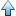. Add a Sine Wave block as the input signal and add a Scope block to capture the input and output of the MATLAB Function block.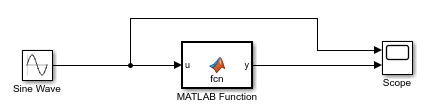4. Run the simulation and open the Scope block to see the effects of the code.

Rename the default function and adjust function arguments to automatically update the block inports, outports, and block icon.

1. Create a new MATLAB Function block and open the MATLAB Function Block Editor.

2. In the function header, change the text to:

`function [x y] = test_function(u1, u2)`

3. Return to the model window. The name on the block icon is now `test_function`, and the block has two input ports for u1 and u2, and two output ports x and y.

Add function arguments and content to the MATLAB Function block by using the Ports and Data Manager.

1. Create a new MATLAB Function block and open the MATLAB Function Block Editor.

2. Click the Ports and Data Manager button.

3. In the Ports and Data Manager window, click Add > Data.

4. To adjust the data properties, click the new data.

You can add breakpoints to debug MATLAB Function blocks.

1. Create a new MATLAB Function block and open the MATLAB Function Block Editor.

2. Enter the following code:

```function [mult,add,subtract] = number_operations(u1,u2) add = u1 + u2; subtract = u1 - u2; mult = u1 * u2; ```

3. Click line numbers 3 and 4 to add two breakpoints. The line numbers highlight in red.

4. Run the simulation. The simulation stops at the first breakpoint. Click the button to simulate until the next breakpoint. If the last breakpoint has been reached, click to advance to the next time step.

Run a report on your MATLAB function code to find information on variables and help detect warnings and errors.

1. Create a new MATLAB Function block and open the MATLAB Function Block Editor.

2. Enter the following code:

```function [mult,add,subtract] = number_operations(u1,u2) add = u1 + u2; subtract = u1 - u2; mult = u1 * u2; ```

3. Click the Function Report button.

4. The report lists variables in the Variables tab at the bottom. Click any of the variable names to highlight instances where the variable appears in the code.

5. In the function code, point to or click any variable or expression to see a dialog box with more information.

## Parameters

Prepare

Launches the Ports and Data Manager. Use the Ports and Data Manager to add, delete, or modify data in MATLAB Function blocks. When you open the Ports and Data Manager, the settings correspond to the MATLAB Function block you are editing with the MATLAB Function Block Editor. For more information, see Define MATLAB Function Block Data.

Navigate

Goes to specified code, including functions and line numbers.

Finds and replaces specified text written in the MATLAB Function Block Editor. You can also use the Ctrl+F keyboard shortcut. This parameter has the same functionality as the MATLAB Editor. For more information, see Find and Replace Text in Files and Go to Location.

Code

Converts selected code to a function. Use this feature on code that is not already contained in a function. You can create a separate file for the function code or you can convert code into a local function.

1. To convert code and save it in a separate m-file, select the code and click Refactor > Convert to Function. The code saves as a m-file and the MATLAB Function Block Editor calls the code by the name you specify. To run the model without error, the m-file must be on the path.

2. To convert code into a local function, select the code and click Refactor > Convert to Local Function. The MATLAB Function Block Editor creates a local function at the bottom of the code and replaces the selected code with a function call to the local function.

Comments out code. Select the lines you want to comment and click the Comment button. The button adds one `%` to the selected lines each time you press it. You can also select the code and use the Ctrl+R command.

Removes comments from code. Select the lines you want to uncomment and click the Uncomment button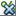. The button removes one `%` from the selected lines each time you press it. You can also select the code and use the Ctrl+T command.

Wraps comments. Select the lines you want to comment and click the Wrap comments button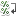.

The MATLAB Function Block Editor does not wrap comments with:

1. Code section titles (comments that begin with `%%`).

2. Long contiguous text, such as URLs.

3. Bulleted list items (text that begins with `*` or `#`) onto the preceding line.

Formats code with smart Indenting. Smart indenting automatically formats code to improve code readability, such as when you use functions or logical statements. To manually deploy smart indenting, select the lines of code and click the Smart Indent button. By default, the MATLAB Function Block Editor automatically uses smart indenting. For more information, see Edit and Format Code.

Increases the code indent by one tab indent. To increase the indent, select the lines of code and click the Increase Indent button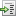.

Decreases the code indent by one tab indent if the code is indented. To decrease the indent, select the lines code and click the Decrease Indent button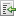.

Builds fi object constructors with a user interface. To open the interface, click the Specify fixed-point data button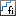. Click the arrow next to the icon to select additional fi constructors. For more information, see Building fimath Object Constructors in a GUI (Fixed-Point Designer). This features requires a Fixed-Point Designer™ license.

Compile

Updates the model and checks for errors. Expand the menu under to select from two options.

• Update Model: Compiles the model and checks for static errors.

For more information, see Update Diagram and Run Simulation and Simulation Phases in Dynamic Systems.

Simulate

Specifies the simulation stop time.

Changes the simulation mode. You can select from Normal, Accelerator or Rapid Accelerator. For more information, see Choosing a Simulation Mode.

Enables fast restart for model simulation. Fast restart can improve performance by preventing compiling. For more information, see Get Started with Fast Restart.

Steps back in simulation. You must configure this option first with the Simulation Stepping Options. If you have not configured these options, the button opens the Simulation Stepping Options window. For more information, see Simulation Stepping Options.

Runs the simulation. You can run a single simulation, or run multiple simulations by creating design studies with Multiple Simulations and selecting Run All from the drop down. Adjust the time pacing by selecting Simulation Pacing from the drop down.

Steps through the simulation at each time step. For more information, see Step Through a Simulation.

Stops the simulation.

Debug Code

Steps over the breakpoint during debugging in the MATLAB Function block. When you press the button, the debugger steps past function calls and does not enter called functions for line-by-line execution. You can use this button only after simulation stops at a breakpoint.

Goes to next line of code during debugging in the MATLAB Function block. If the line calls a local function, step into the first line of the local function. You can use this button only after simulation stops at a breakpoint.

Exits line-by-line function execution during debugging of the current function or local function. If in a local function, the debugger continues to the line following the call to this local function. You can use this button only after simulation stops at a breakpoint.

Report

Opens a report that contains information about the MATLAB Function. You can use this report to find information about variables and expressions in the code. For more information, see MATLAB Function Reports.